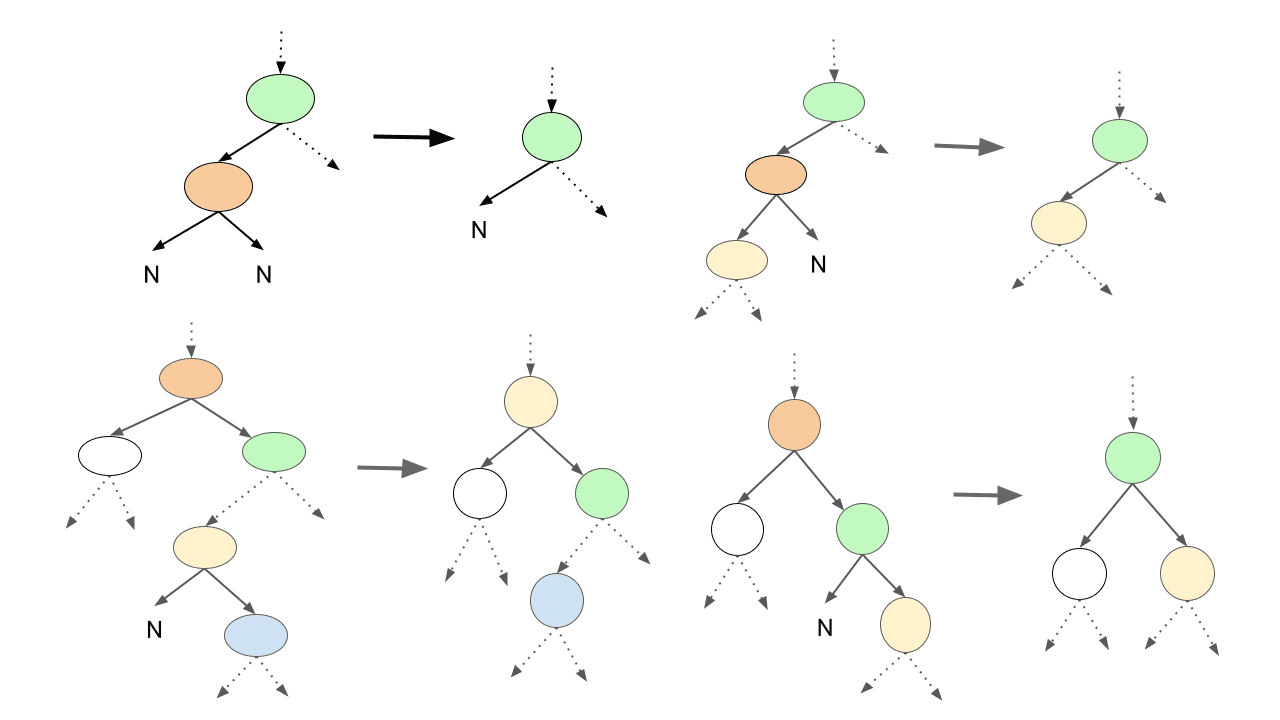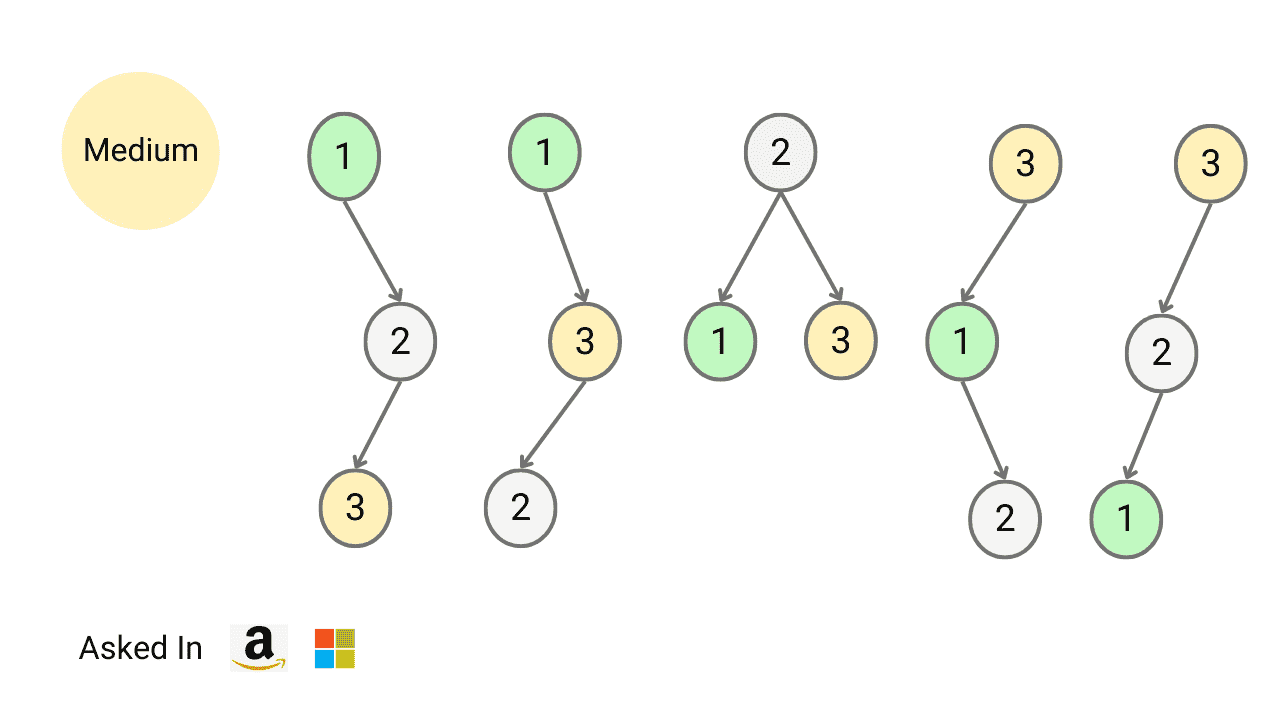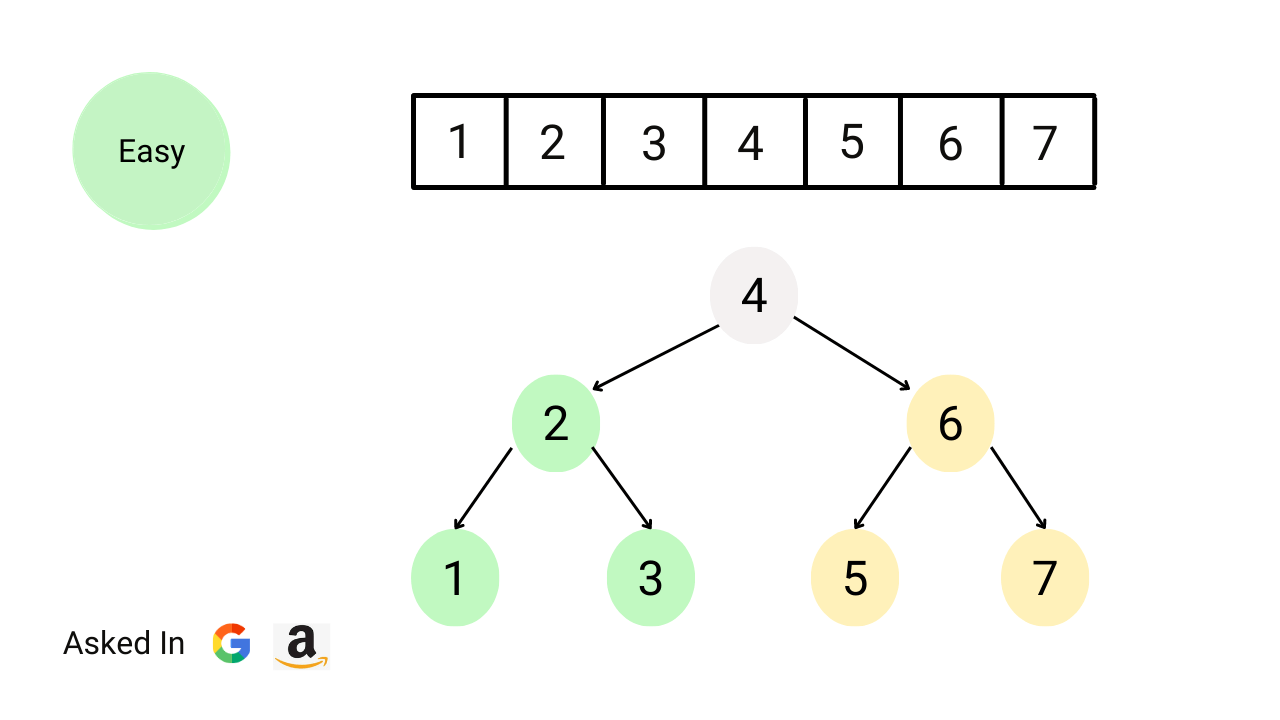# binary-search-treeDelete Node in a Binary Search Tree

Given a binary search tree and a key value. Write a program to delete the given key from the binary search tree and return the updated root node. This is an excellent problem to learn pointer manipulation in binary trees and problem-solving using both iterative and recursive approaches.Count Total Number of Unique Binary Search Trees with n Keys

Write a program to find the number of structurally unique binary search trees (BSTs) that have exactly n nodes, where each node has a unique integer key ranging from 1 to n. In other words, we need to determine the count of all possible BSTs that can be formed using n distinct keys.Convert sorted array to balanced BST

Write a program to convert a sorted array of integers into a balanced binary search tree. Each node in the tree must follow the BST property, and the height of the left and right subtrees should be as close to equal as possible. Note: This is an excellent problem to learn problem-solving using the divide and conquer approach.Minimum Absolute Difference in BST

Given the root of a binary search tree (BST), write a program to find the absolute minimum difference between the values of any two nodes in the tree. Here node values in the tree can be positive, negative, or zero. Note: This is an excellent problem to learn problem-solving using iterative and recursive inorder traversal of a binary tree.Kth Largest Element in BST

Given the root of a BST and an integer k, write a program to find the kth largest value among all the nodes' values in the binary search tree. Note: This is an excellent problem to learn problem solving using recursive and iterative inorder traversal and data structure augmentation (storing extra information inside BST nodes for solving a problem).